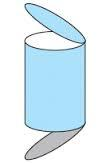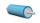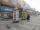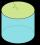# Shell of cylinder

Calculate the content of shell the 1.6 m height cylinder with a base radius of 0.4 m.

Correct result:

S =  4.0212 m2

#### Solution:We would be pleased if you find an error in the word problem, spelling mistakes, or inaccuracies and send it to us. Thank you!#### You need to know the following knowledge to solve this word math problem:

We encourage you to watch this tutorial video on this math problem:

## Next similar math problems:

• RollerCylinder shell has the same content as one of its bases. Cylinder height is 15 dm. What is the radius of the base of the cylinder?
• What isWhat is the height of a cylinder whose surface size is 602.88 cm2 and the content of its shell is 376.8 cm2?
• The cylindrical containerThe container has a cylindrical shape the base diameter 0.8 meters has a content area of the base is equal to the content area of the shell. How many full liters of water can be poured maximally into the container?
• Cylinder - basicsCylinder with base radius r = 45 dm and height h=22 dm. Calculate:
• Rectangular base pyramidCalculate an area of the shell of the pyramid with a rectangular base of 2.8 m and 1.4 m and height 2.5 meters.
• The cylinder 2Find the volume and the lateral area of a cylinder of height 12 inches and a base radius of 4 inches.
• Surface of the cylinderCalculate the surface of the cylinder for which the shell area is Spl = 20 cm2 and the height v = 3.5 cm
• PostersColumn with posters in the form of a cylinder is 2 m high and its diameter is 1.7 m. What is the content area for which it is possible to stick posters?
• Shell area cyThe cylinder has a shell content of 300 cm square, while the height of the cylinder is 12 cm. Calculate the volume of this cylinder.Calculate the surface of a quadrilateral prism according to the input: Area of the diamond base S1 = 2.8 m2, length of the base edge a = 14 dm, height of the prism 1,500 mm.
• Axial sectionAxial section of the cylinder has a diagonal 40 cm. The size of the shell and the base surface are in the ratio 3:2. Calculate the volume and surface area of this cylinder.
• Cylinder surface, volumeThe area of the cylinder surface and the cylinder jacket are in the ratio 3: 5. The height of the cylinder is 5 cm shorter than the radius of the base. Calculate surface area and volume of the cylinder.
• Surface and volumeCalculate the surface and volume of a cylinder whose height is 8 dm and the radius of the base circle is 2 dm
• Cylinder surface areaVolume of a cylinder whose height is equal to the radius of the base is 678.5 dm3. Calculate its surface area.
• Curved surface area CSAA cylinder 5cm high has a base radius(7/2) cm. Calculate the curved surface area.
• Height as diameter of baseThe rotary cylinder has a height equal to the base diameter and the surface of 471 cm2. Calculate the volume of a cylinder.
• Equilateral cylinderEquilateral cylinder (height = base diameter; h = 2r) has a volume of V = 199 cm3 . Calculate the surface area of the cylinder.